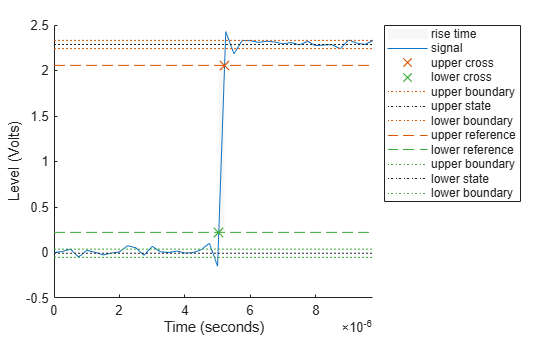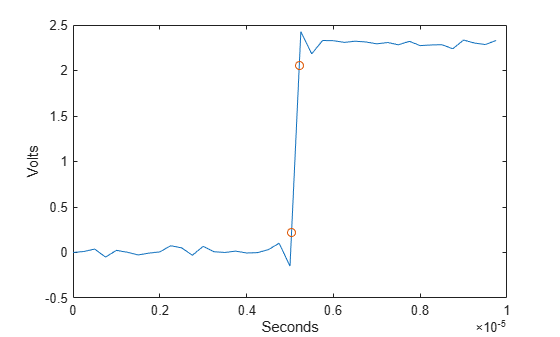Documentation

# risetime

Rise time of positive-going bilevel waveform transitions

## Syntax

``R = risetime(X)``
``R = risetime(X,FS)``
``R = risetime(X,T)``
``````[R,LT,UT] = risetime(___)``````
``````[R,LT,UT,LL,UL] = risetime(___)``````
``[___] = risetime(___,Name,Value)``
`` risetime(___)``

## Description

example

````R = risetime(X)` returns a vector, `R`, containing the time each transition of the input bilevel waveform, `X`, takes to cross from the 10% to 90% reference levels. To determine the transitions, `risetime` estimates the state levels of the input waveform by a histogram method. `risetime` identifies all regions that cross the upper-state boundary of the low state and the lower-state boundary of the high state. The low-state and high-state boundaries are expressed as the state level plus or minus a multiple of the difference between the state levels. See State-Level Tolerances. Because `risetime` uses interpolation, `R` may contain values that do not correspond to sampling instants of the bilevel waveform, `X`.```
````R = risetime(X,FS)` specifies the sample rate in hertz. The sample rate determines the sample instants corresponding to the elements in `X`. The first sample instant in `X` corresponds to t=0. Because `risetime` uses interpolation, `R` may contain values that do not correspond to sampling instants of the bilevel waveform, `X`.```
````R = risetime(X,T)` specifies the sample instants, `T`, as a vector with the same number of elements as `X`.```
``````[R,LT,UT] = risetime(___)``` returns vectors, `LT` and `UT`, whose elements correspond to the time instants where `X` crosses the lower- and upper-percent reference levels. ```

example

``````[R,LT,UT,LL,UL] = risetime(___)``` returns the levels, `LL` and `UL`, that correspond to the lower- and upper-percent reference levels.```

example

````[___] = risetime(___,Name,Value)` returns the rise times with additional options specified by one or more `Name,Value` pair arguments.```
```` risetime(___)` plots the signal and darkens the regions of each transition where rise time is computed. The plot marks the lower and upper crossings and the associated reference levels. The state levels and the corresponding associated lower- and upper-state boundaries are also plotted.```

## Examples

collapse all

Determine the rise time in samples for a 2.3 V clock waveform.

Load the 2.3 V clock data. Determine the rise time in samples. Use the default 10% and 90% percent reference levels.

```load('transitionex.mat','x') R = risetime(x)```
```R = 0.7120 ```

The rise time is less than 1, indicating that the transition occurred in a fraction of a sample. Plot the data and annotate the rise time.

`risetime(x);`Determine the rise time in a 2.3 V clock waveform sampled at 4 MHz. Compute the rise time using the 20% and 80% reference levels.

Load the 2.3 V clock data with sampling instants. Determine the rise time using the 20% and 80% reference levels. Plot the annotated waveform.

```load('transitionex.mat','x','t') risetime(x,'PercentReferenceLevels',[20 80])``````ans = 0.5340 ```

Determine the rise time, reference-level instants, and reference levels in a 2.3 V clock waveform sampled at 4 MHz.

Load the 2.3 V clock waveform along with the sampling instants.

`load('transitionex.mat','x','t')`

Determine the rise time, reference-level instants, and reference levels.

`[R,lt,ut,ll,ul] = risetime(x,t);`

Plot the waveform with the lower- and upper-reference levels and reference-level instants. Show that the rise time is the difference between the upper- and lower-reference level instants.

```plot(t,x) xlabel('seconds') ylabel('Volts') hold on plot([lt ut],[ll ul],'o') hold off````fprintf('Rise time is %g seconds.',ut-lt)`
```Rise time is 1.78e-07 seconds. ```

## Input Arguments

collapse all

Bilevel waveform, specified as a real-valued vector.

Sample rate, specified in as a positive real integer in Hz.

Sample instants, specified as a vector. The length of `T` must equal the length of the bilevel waveform `X`.

### Name-Value Pair Arguments

Specify optional comma-separated pairs of `Name,Value` arguments. `Name` is the argument name and `Value` is the corresponding value. `Name` must appear inside quotes. You can specify several name and value pair arguments in any order as `Name1,Value1,...,NameN,ValueN`.

Reference levels as a percentage of the waveform amplitude, specified as the comma-separated pair consisting of `'PercentReferenceLevels'`and a two-element positive row vector. The elements of the row vector correspond to the lower and upper percent reference levels. The high state level is defined to be 100 percent and the low state level is defined to be 0 percent. See Percent Reference Levels for more details.

Low and high state levels, specified as the comma-separated pair consisting of `'StateLevels'` and a two-element positive row vector. The first and second elements of the vector correspond to the low and high state levels.

Lower- and upper-state boundaries, specified as the comma-separated pair consisting of `'Tolerance'` and a real positive scalar as a percentage value. See State-Level Tolerances for more information on this name-value pair.

## Output Arguments

collapse all

Duration of positive-going transition, returned as a vector. If you specify the sample rate `Fs` or the sample instants `T` rise times are in seconds. If you do not specify a sample rate or sample instants, rise times are in samples.

Lower reference-level crossing instants, returned as a vector. The vector `LT` contains the time instants when the positive-going transition crosses the lower reference level. By default, the lower reference level is the 10% reference level. You can change the default reference levels by specifying the `'PercentReferenceLevels'` name-value pair.

Upper reference-level crossing instants, returned as a vector. The vector `UT` contains the time instants when the positive-going transition crosses the upper reference level. By default, the lower reference level is the 90% reference level. You can change the default reference levels by specifying the `'PercentReferenceLevels'` name-value pair.

Lower reference-level in waveform amplitude units, returned as a real numeric scalar. `LL` is a vector containing the waveform values corresponding to the lower reference level in each positive-going transition. By default, the lower reference-level is the 10% reference level. You can change the default reference-levels by specifying the `'PercentReferenceLevels'` name-value pair.

Upper reference-level in waveform amplitude units, returned as a real numeric scalar. `UL` is a vector containing the waveform values corresponding to the upper reference level in each positive-going transition. By default, the upper reference level is 10%. You can change the default reference levels by specifying the `'PercentReferenceLevels'` name-value pair.

collapse all

### Positive-Going Transition

A positive-going transition in a bilevel waveform is a transition from the low-state level to the high-state level. A positive-polarity (positive-going) pulse has a terminating state more positive than the originating state. If the waveform is differentiable in the neighborhood of the transition, an equivalent definition is a transition with a positive first derivative. This figure shows a positive-going transition.The amplitude values of the waveform do not appear because a positive-going transition does not depend on the actual waveform values. A positive-going transition is defined by the direction of the transition.

### Percent Reference Levels

If S1 is the low state, S2 is the high state, and U is the upper-percent reference level. The waveform value corresponding to the upper percent reference level is

`${S}_{1}+\frac{U}{100}\left({S}_{2}-{S}_{1}\right).$`

If L is the lower-percent reference level, the waveform value corresponding to the lower percent reference level is

`${S}_{1}+\frac{L}{100}\left({S}_{2}-{S}_{1}\right).$`

### State-Level Tolerances

Each state level can have associated lower- and upper-state boundaries. These state boundaries are defined as the state level plus or minus a scalar multiple of the difference between the high state and the low state. To provide a useful tolerance region, the scalar is typically a small number such as 2/100 or 3/100. In general, theregion for the low state is defined as

``

whereis the low-state level andis the high-state level. Replace the first term in the equation withto obtain thetolerance region for the high state.

The following figure illustrates lower and upper 2% state boundaries (tolerance regions) for a positive-polarity bilevel waveform. The red dashed lines indicate the estimated state levels.## References

 IEEE® Standard on Transitions, Pulses, and Related Waveforms, IEEE Standard 181, 2003, pp. 15–17.